Скачать презентацию Machine Learning based on Attribute Interactions Aleks Jakulin

• Количество слайдов: 24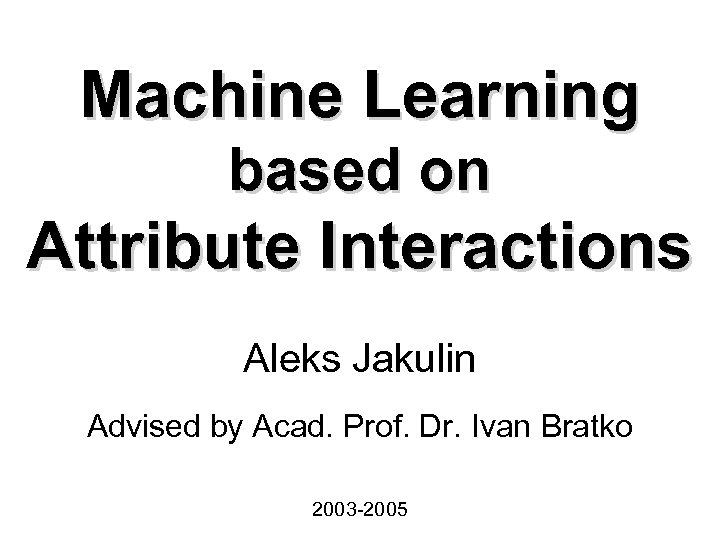Machine Learning based on Attribute Interactions Aleks Jakulin Advised by Acad. Prof. Dr. Ivan Bratko 2003 -2005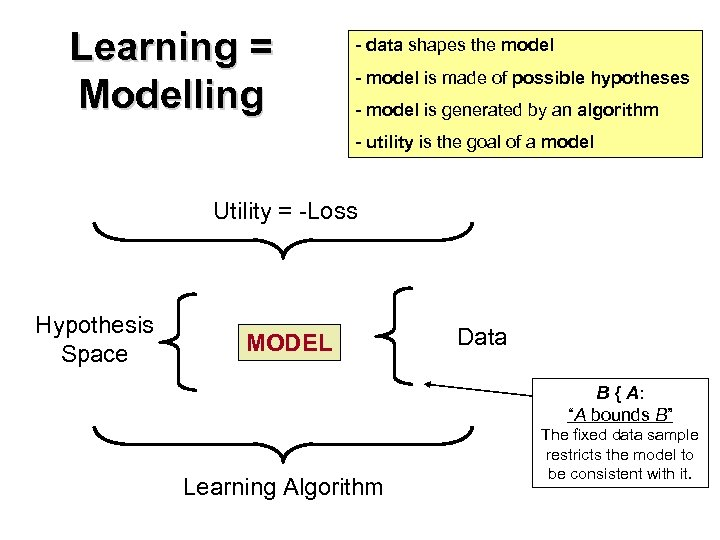Learning = Modelling - data shapes the model - model is made of possible hypotheses - model is generated by an algorithm - utility is the goal of a model Utility = -Loss Hypothesis Space MODEL Data B { A: “A bounds B” Learning Algorithm The fixed data sample restricts the model to be consistent with it.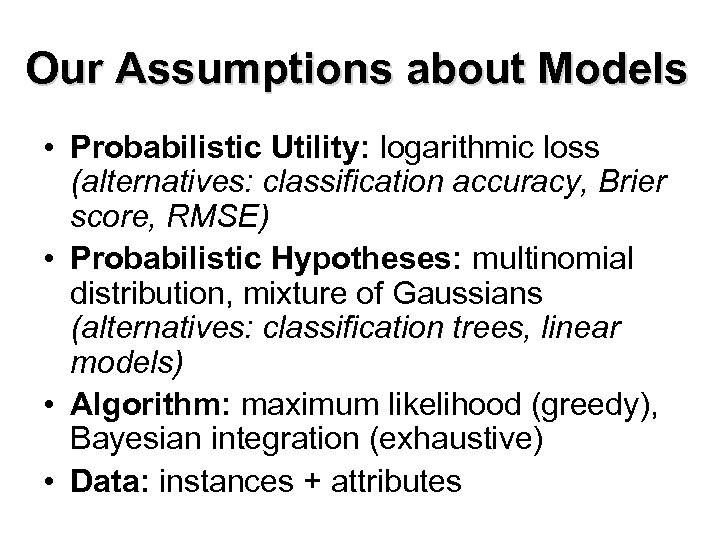Our Assumptions about Models • Probabilistic Utility: logarithmic loss (alternatives: classification accuracy, Brier score, RMSE) • Probabilistic Hypotheses: multinomial distribution, mixture of Gaussians (alternatives: classification trees, linear models) • Algorithm: maximum likelihood (greedy), Bayesian integration (exhaustive) • Data: instances + attributes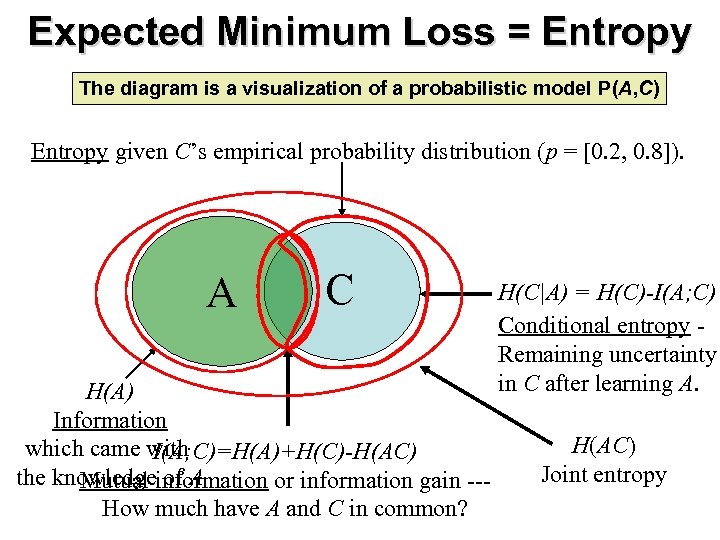Expected Minimum Loss = Entropy The diagram is a visualization of a probabilistic model P(A, C) Entropy given C’s empirical probability distribution (p = [0. 2, 0. 8]). A C H(A) Information which came with I(A; C)=H(A)+H(C)-H(AC) the knowledgeinformation or information gain --Mutual of A How much have A and C in common? H(C|A) = H(C)-I(A; C) Conditional entropy Remaining uncertainty in C after learning A. H(AC) Joint entropy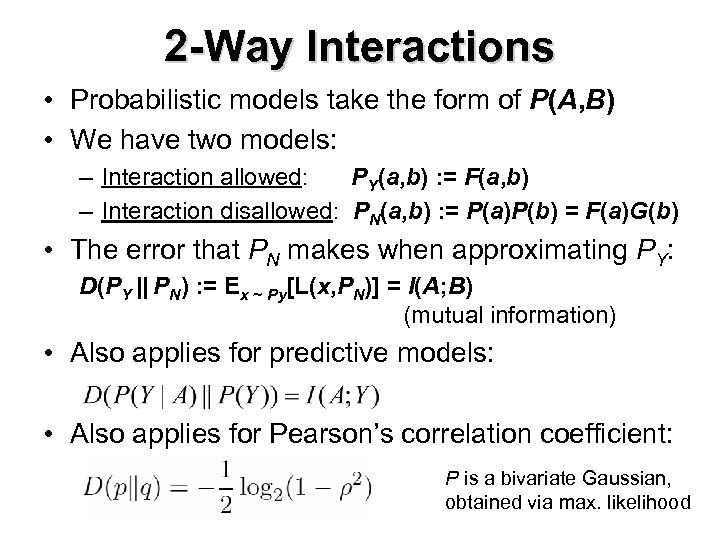2 -Way Interactions • Probabilistic models take the form of P(A, B) • We have two models: – Interaction allowed: PY(a, b) : = F(a, b) – Interaction disallowed: PN(a, b) : = P(a)P(b) = F(a)G(b) • The error that PN makes when approximating PY: D(PY || PN) : = Ex ~ Py[L(x, PN)] = I(A; B) (mutual information) • Also applies for predictive models: • Also applies for Pearson’s correlation coefficient: P is a bivariate Gaussian, obtained via max. likelihood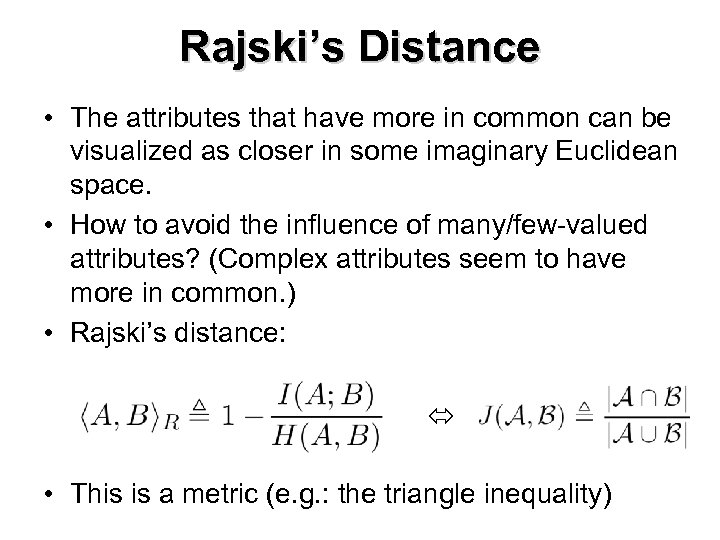Rajski’s Distance • The attributes that have more in common can be visualized as closer in some imaginary Euclidean space. • How to avoid the influence of many/few-valued attributes? (Complex attributes seem to have more in common. ) • Rajski’s distance: • This is a metric (e. g. : the triangle inequality)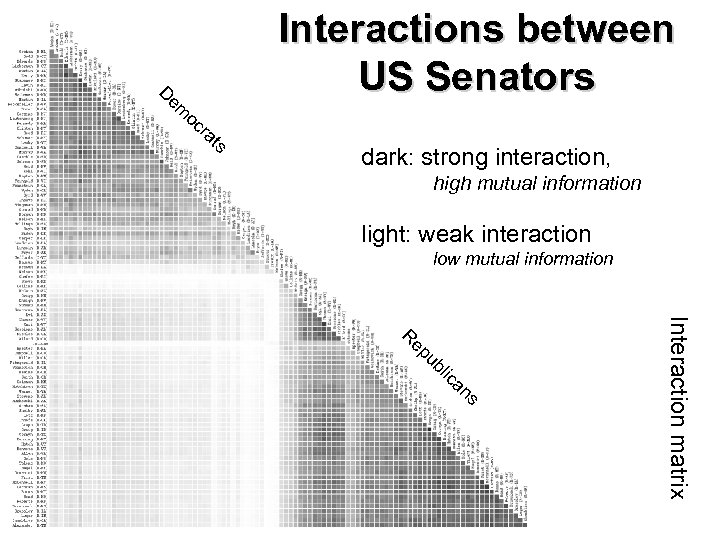Interactions between US Senators D em oc ra ts dark: strong interaction, high mutual information light: weak interaction low mutual information ep ub lic an s Interaction matrix R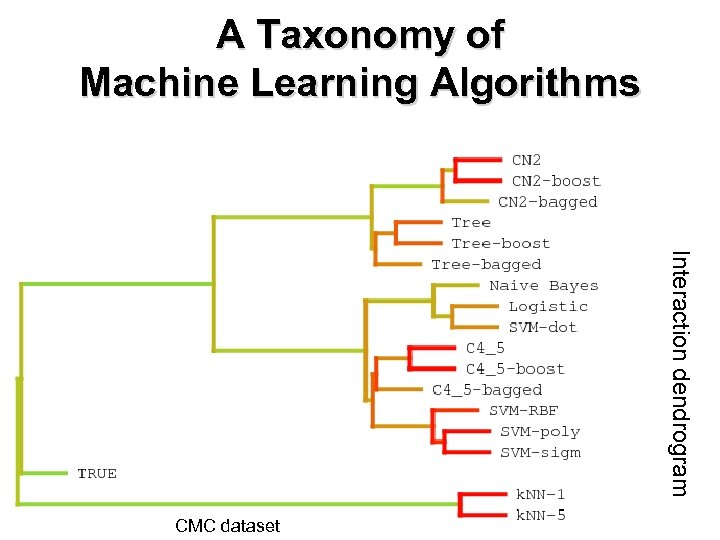A Taxonomy of Machine Learning Algorithms Interaction dendrogram CMC dataset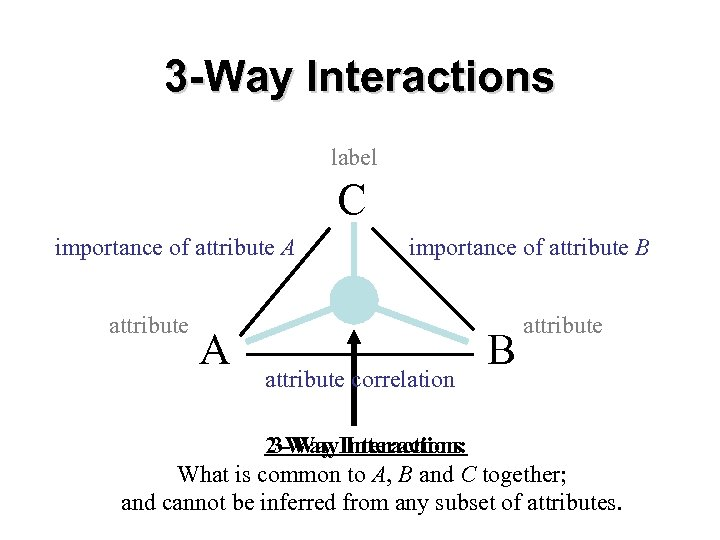3 -Way Interactions label C importance of attribute A importance of attribute B attribute correlation B attribute 3 -Way Interaction: 2 -Way Interactions What is common to A, B and C together; and cannot be inferred from any subset of attributes.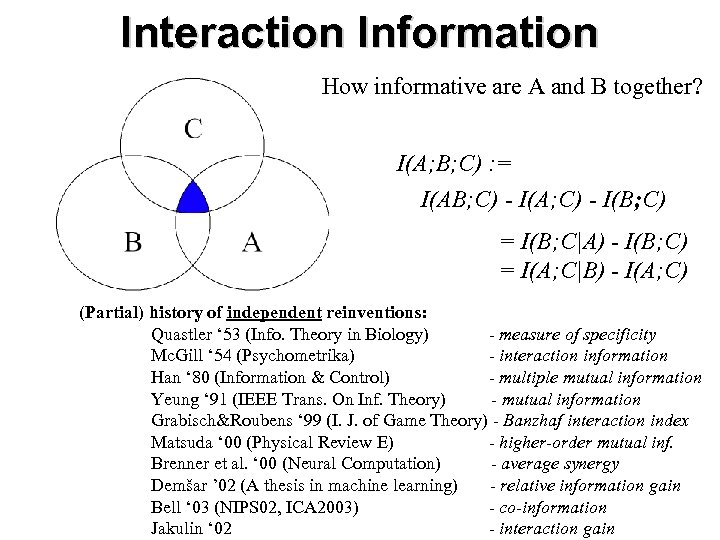Interaction Information How informative are A and B together? I(A; B; C) : = I(AB; C) - I(A; C) - I(B; C) = I(B; C|A) - I(B; C) = I(A; C|B) - I(A; C) (Partial) history of independent reinventions: Quastler ‘ 53 (Info. Theory in Biology) - measure of specificity Mc. Gill ‘ 54 (Psychometrika) - interaction information Han ‘ 80 (Information & Control) - multiple mutual information Yeung ‘ 91 (IEEE Trans. On Inf. Theory) - mutual information Grabisch&Roubens ‘ 99 (I. J. of Game Theory) - Banzhaf interaction index Matsuda ‘ 00 (Physical Review E) - higher-order mutual inf. Brenner et al. ‘ 00 (Neural Computation) - average synergy Demšar ’ 02 (A thesis in machine learning) - relative information gain Bell ‘ 03 (NIPS 02, ICA 2003) - co-information Jakulin ‘ 02 - interaction gain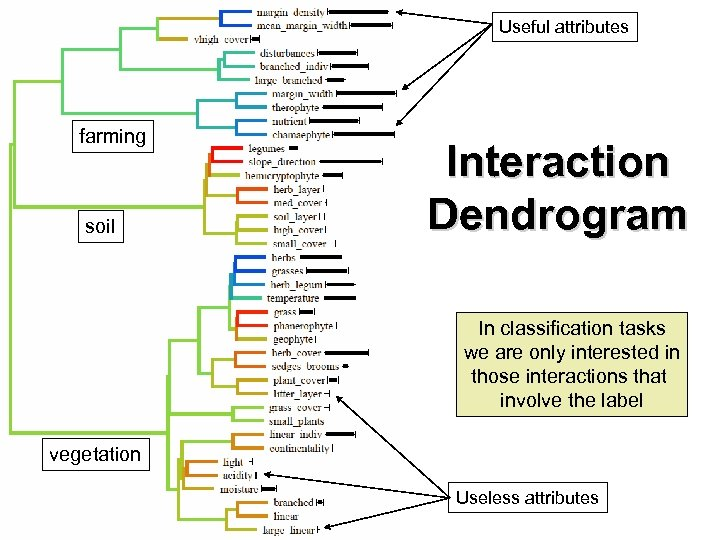Useful attributes farming soil Interaction Dendrogram In classification tasks we are only interested in those interactions that involve the label vegetation Useless attributes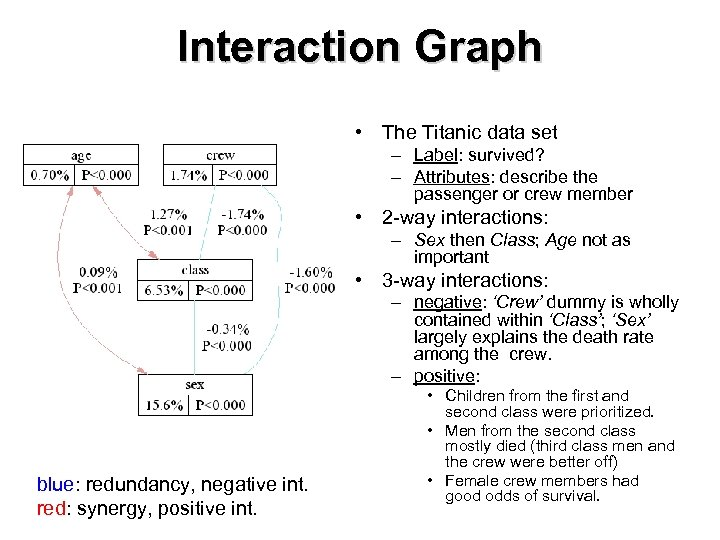Interaction Graph • The Titanic data set – Label: survived? – Attributes: describe the passenger or crew member • 2 -way interactions: – Sex then Class; Age not as important • 3 -way interactions: – negative: ‘Crew’ dummy is wholly contained within ‘Class’; ‘Sex’ largely explains the death rate among the crew. – positive: blue: redundancy, negative int. red: synergy, positive int. • Children from the first and second class were prioritized. • Men from the second class mostly died (third class men and the crew were better off) • Female crew members had good odds of survival.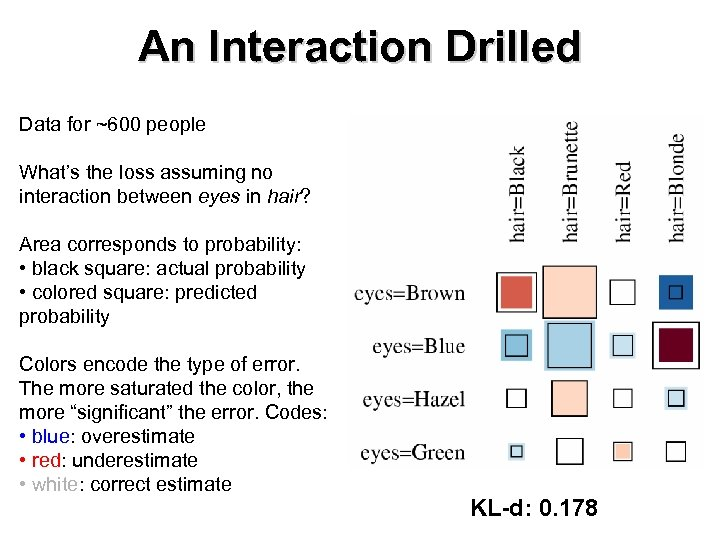An Interaction Drilled Data for ~600 people What’s the loss assuming no interaction between eyes in hair? Area corresponds to probability: • black square: actual probability • colored square: predicted probability Colors encode the type of error. The more saturated the color, the more “significant” the error. Codes: • blue: overestimate • red: underestimate • white: correct estimate KL-d: 0. 178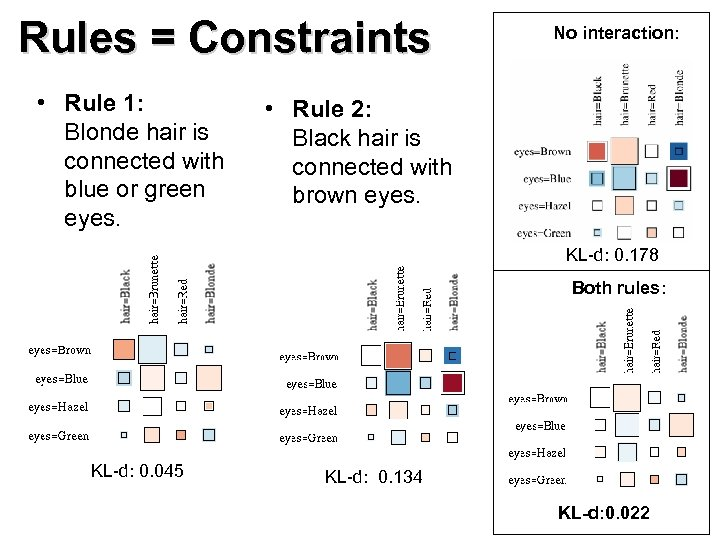Rules = Constraints • Rule 1: Blonde hair is connected with blue or green eyes. No interaction: • Rule 2: Black hair is connected with brown eyes. KL-d: 0. 178 Both rules: KL-d: 0. 045 KL-d: 0. 134 KL-d: 0. 022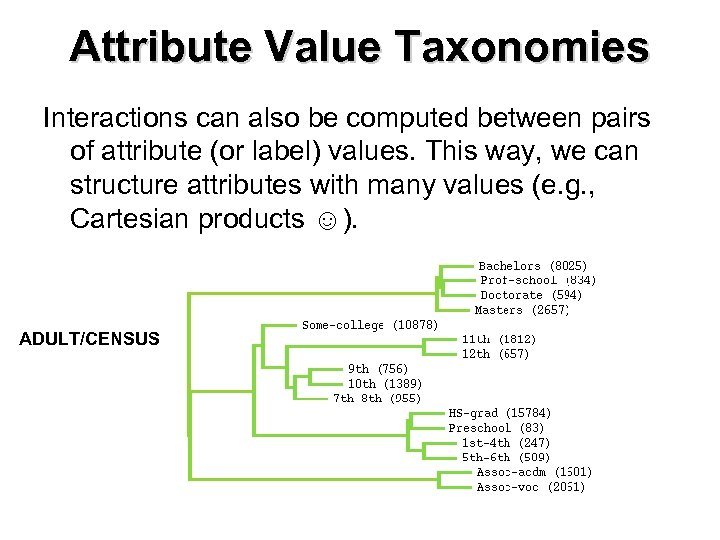Attribute Value Taxonomies Interactions can also be computed between pairs of attribute (or label) values. This way, we can structure attributes with many values (e. g. , Cartesian products ☺). ADULT/CENSUS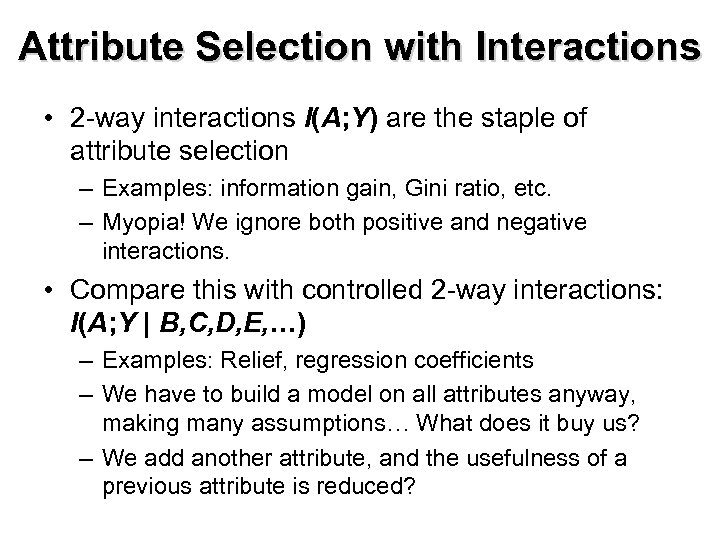Attribute Selection with Interactions • 2 -way interactions I(A; Y) are the staple of attribute selection – Examples: information gain, Gini ratio, etc. – Myopia! We ignore both positive and negative interactions. • Compare this with controlled 2 -way interactions: I(A; Y | B, C, D, E, …) – Examples: Relief, regression coefficients – We have to build a model on all attributes anyway, making many assumptions… What does it buy us? – We add another attribute, and the usefulness of a previous attribute is reduced?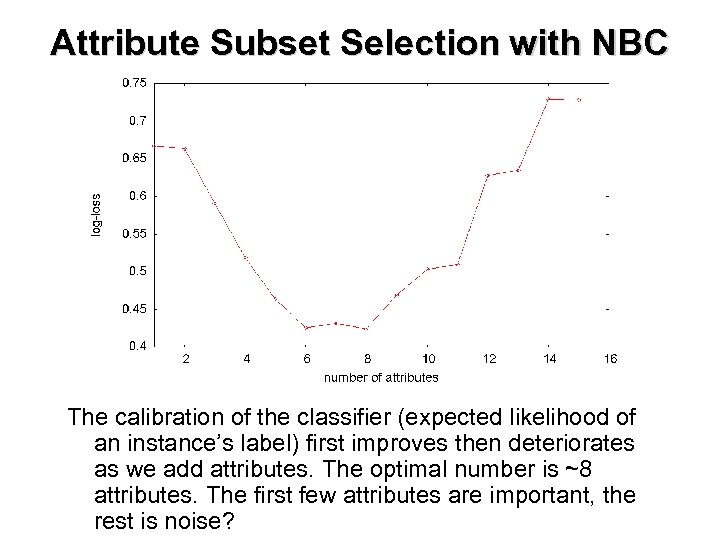Attribute Subset Selection with NBC The calibration of the classifier (expected likelihood of an instance’s label) first improves then deteriorates as we add attributes. The optimal number is ~8 attributes. The first few attributes are important, the rest is noise?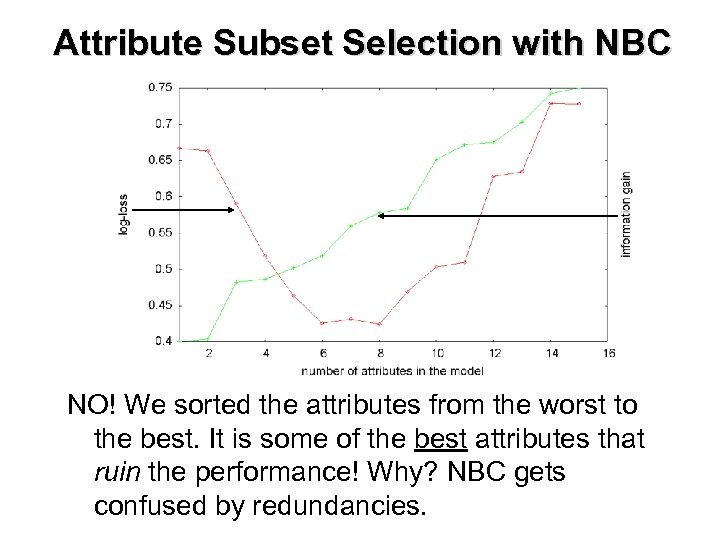Attribute Subset Selection with NBC NO! We sorted the attributes from the worst to the best. It is some of the best attributes that ruin the performance! Why? NBC gets confused by redundancies.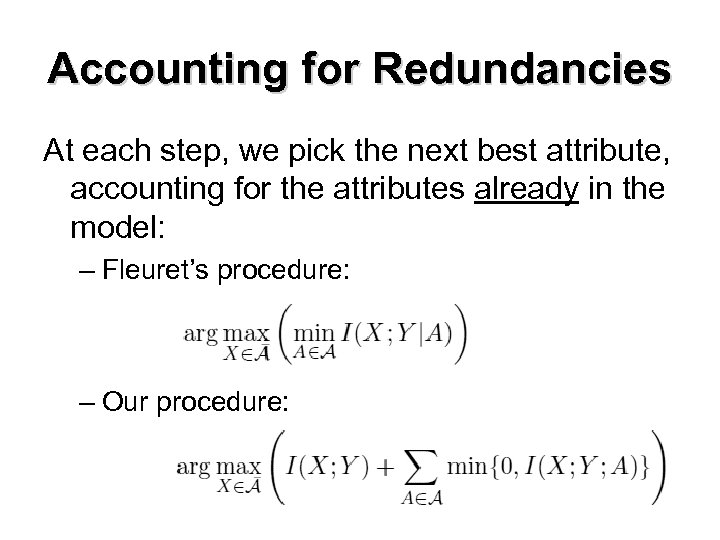Accounting for Redundancies At each step, we pick the next best attribute, accounting for the attributes already in the model: – Fleuret’s procedure: – Our procedure: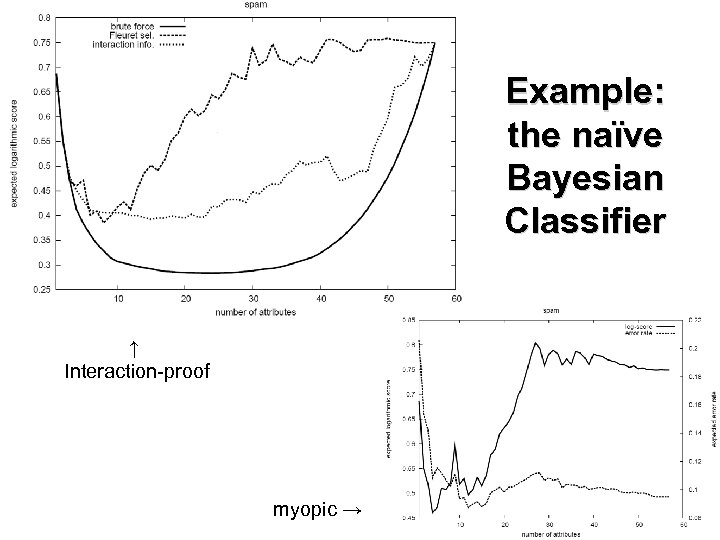Example: the naïve Bayesian Classifier ↑ Interaction-proof myopic →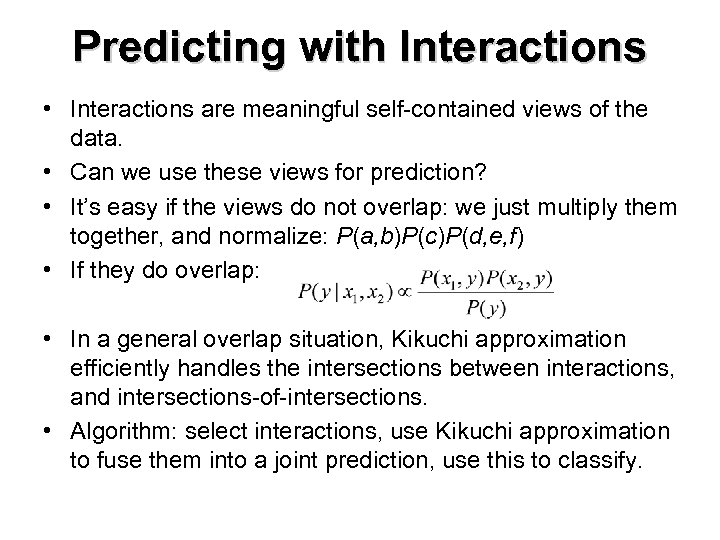Predicting with Interactions • Interactions are meaningful self-contained views of the data. • Can we use these views for prediction? • It’s easy if the views do not overlap: we just multiply them together, and normalize: P(a, b)P(c)P(d, e, f) • If they do overlap: • In a general overlap situation, Kikuchi approximation efficiently handles the intersections between interactions, and intersections-of-intersections. • Algorithm: select interactions, use Kikuchi approximation to fuse them into a joint prediction, use this to classify.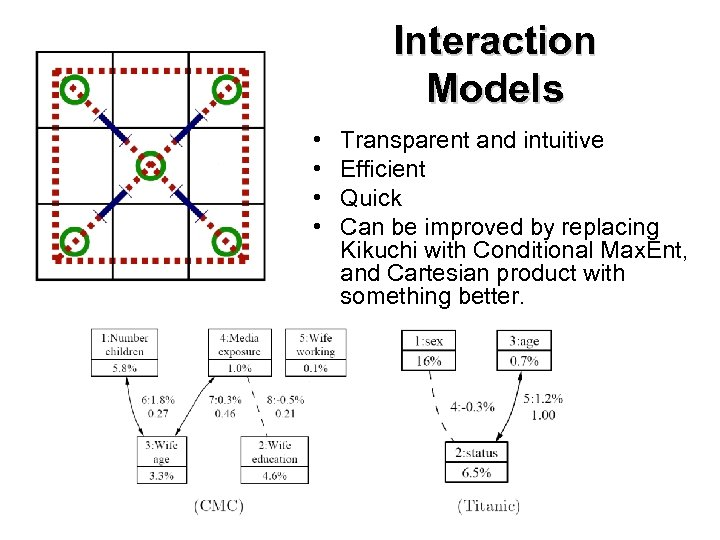Interaction Models • • Transparent and intuitive Efficient Quick Can be improved by replacing Kikuchi with Conditional Max. Ent, and Cartesian product with something better.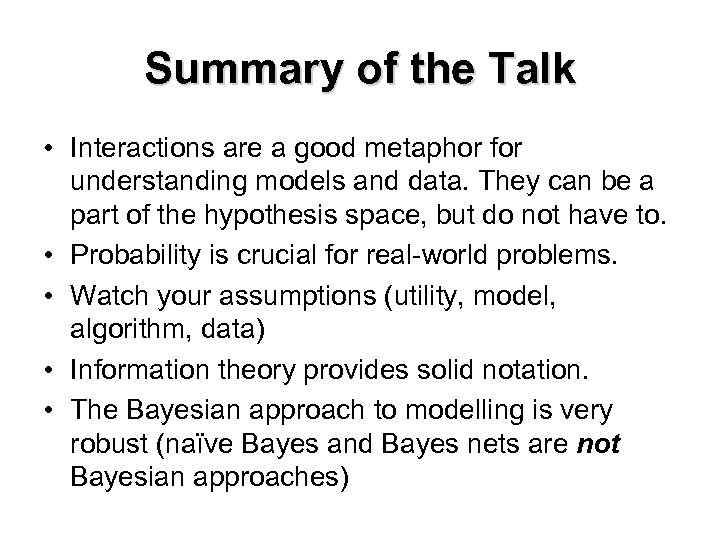Summary of the Talk • Interactions are a good metaphor for understanding models and data. They can be a part of the hypothesis space, but do not have to. • Probability is crucial for real-world problems. • Watch your assumptions (utility, model, algorithm, data) • Information theory provides solid notation. • The Bayesian approach to modelling is very robust (naïve Bayes and Bayes nets are not Bayesian approaches)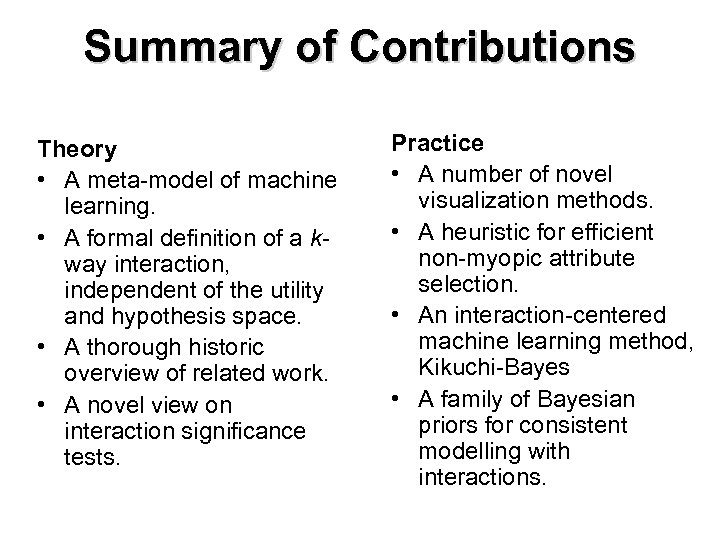Summary of Contributions Theory • A meta-model of machine learning. • A formal definition of a kway interaction, independent of the utility and hypothesis space. • A thorough historic overview of related work. • A novel view on interaction significance tests. Practice • A number of novel visualization methods. • A heuristic for efficient non-myopic attribute selection. • An interaction-centered machine learning method, Kikuchi-Bayes • A family of Bayesian priors for consistent modelling with interactions.